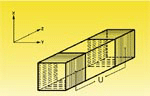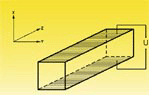AO & EO Crystals

Electro-Optic Crystals

When an electric field (E) is applied to an electro-optic (E-O) crystal, the refractive index of E-O crystal will change linearly to electric field. The phenomenon is called linear electro-optic effect. For KD*P crystal, for example, the change of the refractive index (Dn) is Dn = 0.5n3or63E if both the directions of light propagation and electric field are along the z-axis, where nois refractive index without electric field and r63 is electro-optic coefficient of KD*P.

If a linearly polarized light passes through an E-O crystal, the phase retardation (G) will be induced by Dn to G = 2pDnL, where L is crystal length, for KD*P, again as an example, G = pLn3or63E/l. It is clear that the phase of light will change together with electric field (E). This is called electro-optic phase modulation. If two crossed polarizers are placed at input and output ends of E-O crystal separately, the output intensity of light will be I = I0sin2(G/2), where I0 is input intensity. That means the intensity or amplitude of light can also be modulated by electric field. This is called amplitude modulation.

There are two kinds of E-O modulations. One is longitudinal E-O modulation if the directions of electric field and light propagation are the same. The KDP isomorphic crystals are normally used in this scheme. If the directions of electric field and light propagation are perpendicular, it is called transverse E-O modulation. The LiNbO3, MgO:LiNbO3, ZnO:LiNbO3, BBO and KTP crystals are usually employed in this scheme.

The half-wave voltage (Vp) is defined as the voltage at G = p, for example, Vp=l/(2no3r63)for KD*P and Vp=ld/(2no3r22L) for LiNbO3, where lis light wavelength and d is the distance between the electrodes.Longitudinal Modulatortransverse modulator

 Acousto-Optic Crystals A piezoelectric transducer is bonded to an anisotropic medium. When an electrical signal is applied to this transducer to generate ultrasonic waves which are propagated through the medium, a three dimensional grating was formed by virtue of refractive index variation associated with the traveling acoustic wave. The incident beam passing through the medium will be coupled into several symmetrical diffraction orders, just as in a two-dimensional diffraction grating. The following properties are required for acousto-optic materials: 1. High optical transparency over wavelength range of interest  2. Chemical stability and mechanical durability  3. Large single crystal with high optical quality  4. High acousto-optic figure of merit  5. Low acoustic attenuation of high frequency  6. Low temperature coefficient of physical constants TeO2 and PbMoO4 crystals have been proven to be good acousto-optic media and currently used for A-D modulator, deflector and tunable filter (AOTF).